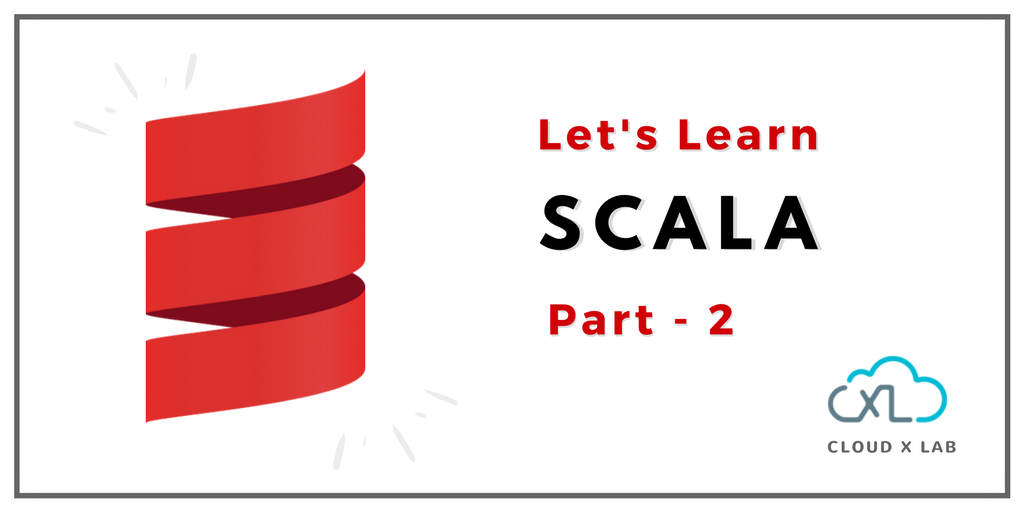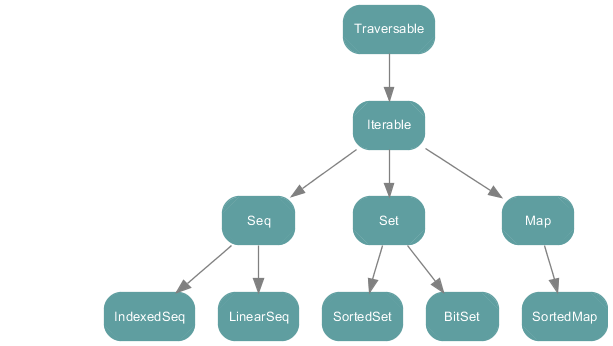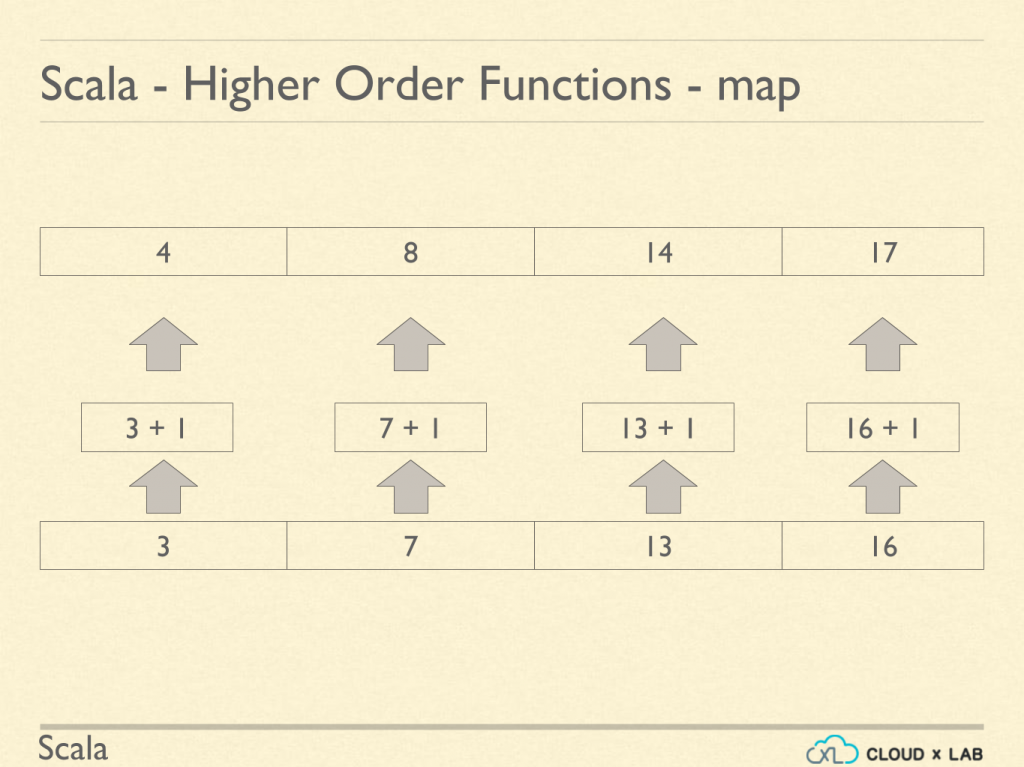# A Simple Tutorial on Scala – Part – 2Welcome back to the Scala tutorial.

This post is the continuation of A Simple Tutorial on Scala – Part – 1

In the Part-1 we learned the following topics on Scala

• Scala Features
• Variables and Methods
• Condition and Loops
• Variables and Type Inference
• Classes and Objects

Keeping up the same pace, we will learn the following topics in the 2nd part of the Scala series.

• Functions Representation
• Collections
• Sequence and Sets
• Tuples and Maps
• Higher Order Functions
• Build Tool – SBT

## Functions Representation

We have already discussed functions. We can write a function in different styles in Scala. The first style is the usual way of defining a function.

```scala> def add(x : int, y : int) : Int = {
return x + y
}```

Please note that the return type is specified as Int.

In the second style, please note that the return type is omitted, also there is no “return” keyword. The Scala compiler will infer the return type of the function in this case.

```scala> def add(x : int, y : int) = { //return type is inferred
x + y //"return" keyword is optional
}```

If the function body has just one statement, then the curly braces are optional. In the third style, please note that there are no curly braces.

`def add(x : Int, y : Int) = x + y`

## Collections Overview

Scala collections provide different ways to store data.

The Scala collections hierarchy represents a structure of collections that can contain data in different ways.At the top is the “traversable”, something that can be traversed. Next in the hierarchy is the iterable. It contains data that can be iterated or looped over. Beyond iterable, we have a Sequence, a set, and a map. The sequence consists of linear Sequence and indexed Sequence.

In Linear Sequence, we iterate over one by one to find the elements that we want. A linked list is an example of linear Sequence. An indexed Sequence gives the ability to directly access the value inside of a sequence.

## Collections – Sequence and Sets

A sequence is an ordered collection of data. Elements in the sequence may or may not be indexed. Examples of sequence are array, list, and vector

### Sequence – Array

Below are the properties of array

• An array contains elements of the same type.
• Arrays are fixed in size and contain an ordered sequence of data.
• Array values are contiguous in memory, which means that the values are stored in consecutive memory addresses.
• Array elements are indexed by position.
`scala> var languages = Array("Ruby", "SQL", "Python")`

In the above code, variable language is an array which contains 3 elements of string type “Ruby”, “SQL” and “Python”.

We can access the array elements by their indexes.

```scala> var languages = Array("Ruby", "SQL", "Python")

Scala> languages(0)
res0: String = Ruby```

Please note that arrays have a zero-based index. Which means to access the first element, languages(0) must be used.

Also, arrays in Scala are mutable. We can change the values at specific indexes.

To iterate over array elements we can use the ‘for’ loop. Let’s iterate over languages array.

```scala> for(x <- languages) {
println(x);
}
Ruby
C++
Python```

As you can see, each element of the languages array gets printed on the screen. The left arrow operator is called generator. We’re iterating over the languages array one by one, assigning the element’s value to x and printing x.

### Sequence – List

List in Scala represents a linked list having elements such that each element has a value and pointer to the next element. These lists have poor performance as data could be located anywhere in the memory.

Compared to an array, a list is very flexible, as you do not have to worry about exceeding the size of the list.

Theoretically, lists are unbounded in size, but practically their size is limited by the amount of memory allocated to the JVM.

Let us do a hands-on and create a list of integers 1, 2 and 3.

`scala> var number_list = List(1, 2, 3)`

To add a new element to the list, type number_list :+ 4

```scala> var number_list = List(1, 2, 3)

scala> number_list :+ 4
res0: List[Int] = List(1,2,3,4)```

As you can see, element 4 is added to the list.

Let us try to change the value at index 1.

```scala> number_list(1) = 7
<console>:13: error: value update is not a member of List[Int]
number_list(1) = 7
^```

We’ve got an error. We can not change the value since lists are immutable.

### Sets

A set in Scala is a bag of data with no duplicates. Also, the ordering is not guaranteed in sets.

Let us create a set with integers 76, 5, 9, 1 and 2.

```scala> var set = Set(76, 5, 9, 1, 2)
set: scala.collection.immutable.Set[Int] = Set(5, 1, 9, 2, 76)```

Let us add 9 to it.

```scala> var set = Set(76, 5, 9, 1, 2)
set: scala.collection.immutable.Set[Int] = Set(5, 1, 9, 2, 76)

scala> set = set + 9
set: scala.collection.immutable.Set[Int] = Set(5, 1, 9, 2, 76)```

Since duplicates are not allowed in sets, 9 is not added to the set.

```scala> var set = Set(76, 5, 9, 1, 2)
set: scala.collection.immutable.Set[Int] = Set(5, 1, 9, 2, 76)

scala> set = set + 20
set: scala.collection.immutable.Set[Int] = Set(5, 20, 1, 9, 2, 76)```

We can see that 20 is added to the set. Note the order of 20. As discussed, set does not guarantee the ordering. set(5) will result in boolean true as 5 is present in the set. set(14) will result in boolean false as 14 is not present in the set.

## Collections – Tuples and Maps

### Tuples

Unlike an array and list, tuples can hold elements of different data types. Let’s create a tuple with elements 14, 45.69 and “Australia”). We can create it either with

```scala> var t = (14, 45.69, "Australia")
t: (Int, Double, String) = (14, 45, 69, Australia)```

or with

```scala> var t = Tuple3(14, 45.69, "Australia")
t: (Int, Double, String) = (14, 45, 69, Australia)```

In the second syntax, we are explicitly specifying that tuple will contain 3 elements.

Tuples can be accessed using a 1-based accessor for each value. To access the first element, type t._1.

```scala> t._1
res0: Int = 14```

Likewise, you can access the third element by t._3

Please note that Tuples are immutable.

### Maps

Scala maps are a collection of key/value pairs. Maps allow indexing values by a specific key for fast access. You can think of a Scala map as a Java Hashmap and Python dictionary.

Let’s do a hands-on on Scala maps. We will start by creating a Colors map that contains key/value pair of two colors and their hex code.

```scala> var colors = Map("red" -> "#FF0000", "yellow" -> "#FFFF00")
```

To see the hex code of color yellow, type colors(“yellow”).

```scala> var colors = Map("red" -> "#FF0000", "yellow" -> "#FFFF00")

scala> colors("yellow")
res0: String = #FFFF00```

As you can see, the hex code of yellow is #FFFF00.

We can add new key/value pairs using += operator. Let’s add color green and its hex value.

`scala> colors += "green" -> "#000000"`

Type colors to see the list of updated key/value pairs.

```scala> colors
res0: scala.collection.immutable.Map(String.String) = Map(red -> #FF0000, yellow -> #FFFF00, green ->
#008000)```

We can also remove key/value pair using -= operator. Let’s remove key “red”.

```scala> colors -= "red"

scala> colors
res0: scala.collection.immutable.Map(String.String) = Map(yellow -> #FFFF00, green -> #008000)```

You can see that key “red” is no more in the map.

Scala provides two types of maps – immutable and mutable. Please note by default, Scala uses the immutable Maps. It means that you can not change the value of a key. If you want to use the mutable maps, import scala.collection.mutable.Map class explicitly

## Higher Order Functions

In Scala, a higher-order function is a function which takes another function as an argument. A higher-order function describes “how” the work is to be done in a collection.

### Higher Order Function – Map

Let’s learn the higher order function map.The map applies the function to each value in the collection and returns a new collection. Say we have a list of integers 3, 7, 13 and 16 and we want to add one to each value in the list. Using higher order map function, we can map over the list and add one to each value. As displayed on the image above, we have a new list with values 4, 8, 14 and 17.

Let’s do a hands-on. Define a list of integers 1, 2 and 3.

`scala> var list = List(1,2,3)`

Let’s add 1 to each element using the map function.

```scala> list.map(x => x + 1)
res0: List[Int] = List(2, 3, 4)```

As you can see, we have a new list with integers 2, 3 and 4

Here map function adds one to every value in the list. Each value in the list has the name “x”. There is another syntax to define the map, where instead of giving a name to every value being used in the function, we use a placeholder underscore to represent the value.

`scala> list.map(_+ 1)`

### Higher Order Function – flatMap

flatMap takes a function as an argument and this function must return a collection. The collection could be empty.

The flatMap is called on a collection. The flatMap applies the function passed to it as an argument on each value in the collection just like map.

The returned collections from each call of function are then merged together to form a new collection.

Let’s understand it with an example

Let’s define a list of languages.

`var list = List("Python", "Go")`

Here, we have a list of strings. A string is nothing but a sequence of characters.

Now, we define a flatMap.

```scala> list.flatMap(lang => lang + "#")
res0: List(Char) = List(P, y, t, h, o, n, #, G, o, #)```

flatMap appends “#” to every string in the list and then flattens down the string to characters. As you can see, # is appended to python and go and then “python#” and “go#” flatten down into the sequence of characters.

We can also define the same function using flatMap(_+ “#”) where the underscore is a placeholder for each value in the list.

So, the size of output collection could be larger than the size of input collection.

While in the case of map the input and output collection were of the same size.

### Higher Order Function – Filter

Let’s learn the higher order function Filter. The filter applies a function to each value in the collection and returns a new collection with values that satisfy a condition specified in the function. Let’s understand it with an example.

We have a list of languages Scala, R, Python, Go and SQL. To filter out languages which contain capital S, we use the following command

```scala> var list = List("Scala", "R", "Python", "Go", "SQL")

scala> list.filter(lang => lang.contains("S"))
res0: List(String) = List(Scala, SQL)```

As you can see we have a new list now with only Scala and SQL as values. Each value in the list has name “lang”

Each of the previous higher order functions returned a new collection after applying the transformation. But at times we do not want the functions to return a new collection. It is a waste of memory resources on JVM if we do not want a return value. Higher order function ‘foreach’ allows us to apply a function to each value of collection without returning a new collection.

Let’s say we have a list of values 1 and 2 and we just want to print each value in the list. We can use foreach function for this as we are not interested in the return value. To use foreach higher order function, type

```scala> var list = List(1,2)
list: List[Int] = List(1,2)

scala> var list.foreach(println)
1
2```

As you can see value 1 and 2 are printed on the screen.

### Higher Order Function – Reduce

Reduce is a very important concept in the MapReduce world. Let’s say we have a list and we want to reduce it to add values together. Let’s understand it with an example.

In our example, reduce adds the first two elements 3 and 7 which results in 10. Then it adds 10 to the next element which is 13 resulting in 23. Then it adds 23 to the next element in the list which is 16.

```scala> var list = List(3, 7, 13, 16)

scala> list.reduce((x, y) => x + y)
res0: Int = 39
```

As you can see, that final result of the reduce function is 39

Here the reduce function takes two values named x and y and adds them.

We can also define the reduce function using list.reduce( + ). Here the two underscores are placeholders for the two values.

## Build Tool – SBT

So far, we have just compiled and run one .scala file. If we are working on a big project containing hundreds of source files, it becomes really tedious to compile these files manually. We’ll then need a build tool to manage the compilation of all these files. SBT is a build tool for Scala and Java projects, similar to Java’s Maven or ant.

SBT is already installed on CloudxLab so you can compile your Scala project directly on it.

Let’s understand how to use SBT to build a Scala project. We’ve provided a sample code on the CloudxLab GitHub repository. Clone the CloudxLab GitHub repository in your home folder in CloudxLab.

Follow the instructions given in the video below.